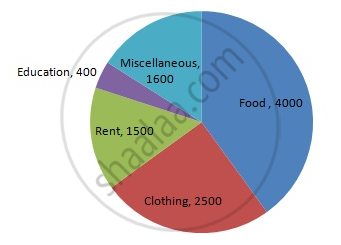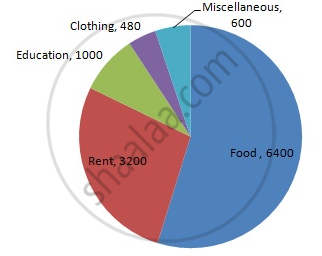Share

# Represent the Following Data by a Pie-diagram: - Mathematics

Course
ConceptCircle Graph Or Pie Chart - Drawing Pie Charts

#### Question

Represent the following data by a pie-diagram:

 Items of expenditure Expenditure Family A Family B Food 4000 6400 Clothing 2500 480 Rent 1500 3200 Education 400 1000 Miscellaneous 1600 600 Total 10000 16000

#### Solution

We know:
Central angle of a component = (component value/sum of component values x 360)
Here the total expenditure of family A = 10000 and family B = 11680

Thus the central angle for each component can be calculated as follows:

 Item Expenditure (Family A) Sector angle (Family A) Expenditure(Family B) Sector angle(Family B) Food 4000 4000/10000  x 360 = 144 6400 6400/11680  x 360 = 197.3 Clothing 2500 2500/10000  x 360 = 90 480 480/11680  x 360 = 14.8 Rent 1500 1500/10000  x 360 = 54 3200 3200/11680  x 360 = 98.6 Education 400 400/10000  x 360 = 14.4 1000 1000/11680  x 360 = 30.8 Miscellaneous 1600 1600/10000  x 360 = 57.6 600 600/11680  x 360 = 18.5

Total expenditure of family A: 10000
Total expenditure of family B: 11680  (not 16000)

Now, the pie chat representing the given data can be constructed by following the steps below:
Step 1 : Draw circle of an appropriate radius.
Step 2 : Draw a vertical radius of the circle drawn in step 1.
Step 3 : Choose the largest central angle. Here the largest central angle is 144o. Draw a sector with the central angle 144o in such a way that one of its radii coincides with the radius drawn in step 2 and another radius is in its counter clockwise direction.
Step 4 : Construct other sectors representing the other items in the clockwise sense in descending order of magnitudes of their central angles.
​Step 5 : Shade the sectors with different colours and label them, as shown as in figure below.​​\

Family AFamily BIs there an error in this question or solution?

#### APPEARS IN

RD Sharma Solution for Mathematics for Class 8 by R D Sharma (2019-2020 Session) (2017 to Current)
Chapter 25: Data Handling-III (Pictorial Representation of Data as Pie Charts or Circle Graphs)
Ex. 25.1 | Q: 12 | Page no. 13

#### Video TutorialsVIEW ALL 

Solution Represent the Following Data by a Pie-diagram: Concept: Circle Graph Or Pie Chart - Drawing Pie Charts.
S